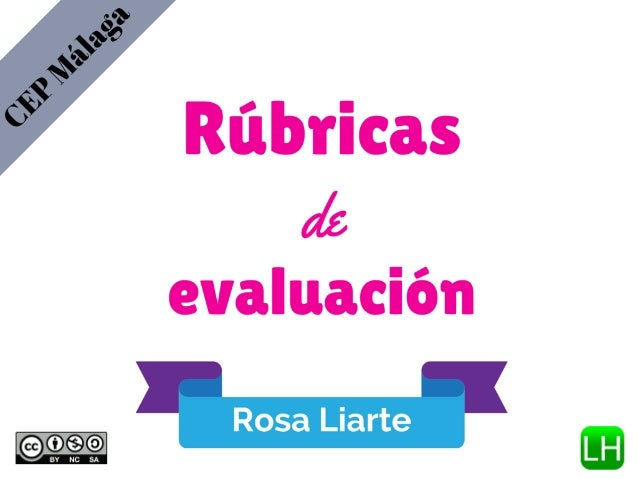Successfully reported this slideshow.Upcoming SlideShare
×

# Rubricas de evaluación en el aula y CoRubrics

633,710 views

Published on

Presentación realizada para la ponencia "Rúbricas de evaluación en el aula y CoRubrics" para el curso "Cultura Evaluativa" del CEP de Málaga

Published in: Education
• Full Name
Comment goes here.

Are you sure you want to Yes No### Rubricas de evaluación en el aula y CoRubrics

1. 1. Profesora de Geografía e Historia y miembro del Equipo Directivo (Secretaria) del IES Nuevo Estación de Cártama (Málaga)
2. 2. ProyectoCartama.es
3. 3. 1947
4. 4. ¿Aprendemos igual que nuestros antepasados? ¿Evaluamos igual?
5. 5. Prensa:Educaciónenel2030
6. 6. Ejemplos de rúbricas
7. 7. Webs con recursos de rúbricas http://bit.ly/1Evd333 http://bit.ly/1Evd72T http://bit.ly/1EvdcUd http://bit.ly/1xXFp2B http://goo.gl/kxDkbr
8. 8. Conceptos 1 2 3 4 50% 0,50 x 1 = 0,5 0,50 x 2 = 1 0,50 x 3 = 1,5 0,50 x 4 = 2 25% 0,25 x 1 = 0,25 0,25 x 2 = 0,5 0,25 x 3 = 0,75 0,25 x 4 = 1 25% 0,25 x 1 = 0,25 0,25 x 2 = 0,5 0,25 x 3 = 0,75 0,25 x 4 = 1
9. 9. Conceptos 1 2 3 4 50% 0,50 x 1 = 0,5 0,50 x 2 = 1 0,50 x 3 = 1,5 0,50 x 4 = 2 25% 0,25 x 1 = 0,25 0,25 x 2 = 0,5 0,25 x 3 = 0,75 0,25 x 4 = 1 25% 0,25 x 1 = 0,25 0,25 x 2 = 0,5 0,25 x 3 = 0,75 0,25 x 4 = 1 Máxima caliﬁcación 4 puntos = 10
10. 10. Conceptos 1 2 3 4 50% 0,50 x 1 = 0,5 0,50 x 2 = 1 0,50 x 3 = 1,5 0,50 x 4 = 2 25% 0,25 x 1 = 0,25 0,25 x 2 = 0,5 0,25 x 3 = 0,75 0,25 x 4 = 1 25% 0,25 x 1 = 0,25 0,25 x 2 = 0,5 0,25 x 3 = 0,75 0,25 x 4 = 1 = 2 puntos (Máximo 4 puntos)
11. 11. Conceptos 1 2 3 4 25% 1 2 3 4 25% 1 2 3 4 25% 1 2 3 4 25% 1 2 3 4 Si aplicamos mismo porcentaje más fácil Máximo 16 puntos = a 10 Mínimo 4 puntos = a 2,5
12. 12. ¡Gracias Jaume!
13. 13. ASÍ ME QUEDA A MI EVALUANDO UN PROYECTO
14. 14. LISTADO DE LOS GRUPOS
15. 15. EL ALUMNADO O PROFE VERÁ ESTO
16. 16. SELECCIONAR ALUMNADO Y ¡A EVALUAR!
17. 17. OBTIENES LOS RESULTADOS AUTOMÁTICAMENTE
18. 18. http://bit.ly/1wuZDKm http://bit.ly/16YDyzd enlaces explicando corubrics su autor Desde su web videotutorial